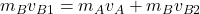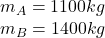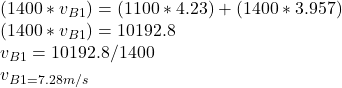## In the “before” part of Fig. 9-60, car A (mass 1100 kg) is stopped at a traffic light when it is rear-ended by car B (mass 1400 kg). Both ca

Question

In the “before” part of Fig. 9-60, car A (mass 1100 kg) is stopped at a traffic light when it is rear-ended by car B (mass 1400 kg). Both cars then slide with locked wheels until the frictional force from the slick road (with a low

in progress 0
5 months 2021-08-19T18:58:15+00:00 2 Answers 74 views 0

## Answers ( )

1. Complete Question:

In the “before” part of Fig. 9-60, car A (mass 1100 kg) is stopped at a traffic light when it is rear-ended by car B (mass 1400 kg). Both cars then slide with locked wheels until the frictional force from the slick road (with a low ?k of 0.15) stops them, at distances dA = 6.1 m and dB = 4.4 m. What are the speeds of (a) car A and (b) car B at the start of the sliding, just after the collision? (c) Assuming that linear momentum is conserved during the collision, find the speed of car B just before the collision.

a) Speed of car A at the start of sliding = 4.23 m/s

b) speed of car B at the start of sliding = 3.957 m/s

c) Speed of car B before the collision = 7.28 m/s

Explanation:

NB: The figure is not provided but all the parameters needed to solve the question have been given.

Let the frictional force acting on car A,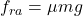…………(1)

Since frictional force is a type of force, we are safe to say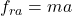…….(2)

Equating (1) and (2)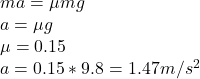a) Speed of A at the start of the sliding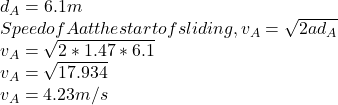b) Speed of B at the start of the sliding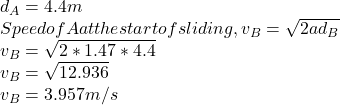Let the speed of car B before collision =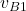Momentum of car B before collision =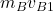Momentum after collision =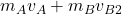Applying the law of conservation of momentum: